# Selina Solutions Concise Mathematics Class 6 Chapter 7: Number Line Exercise 7(A)

Selina Solutions Concise Mathematics Class 6 Chapter 7 Number Line Exercise 7(A) contains problems on integers that help students to grasp the concepts covered in-depth. The exercise wise problems consist of arranging the integers in ascending and descending order, on a given Number Line. After thorough research, solutions are curated by our expert teachers, with the intention to help students in boosting their problem solving abilities. Students, who find obstacles in solving textbook questions, can make use of Selina Solutions, which are presented as per their understanding capacity. This also helps them in solving the tricky questions with ease, that would appear in examination. Students can download the Selina Solutions Concise Mathematics Class 6 Chapter 7 Number Line Exercise 7(A) in PDF format, from the links provided below

## Selina Solutions Concise Mathematics Class 6 Chapter 7: Number Line Exercise 7(A) Download PDF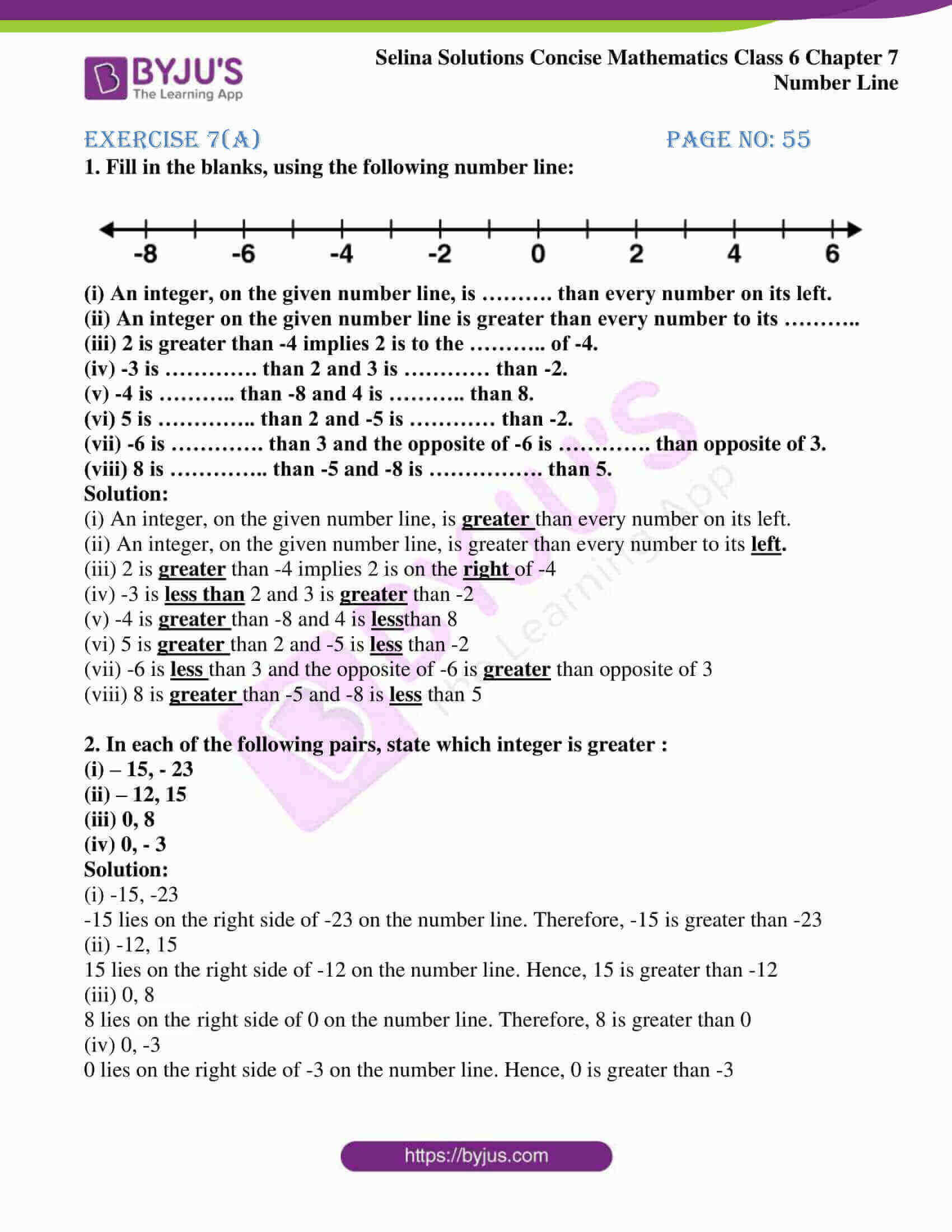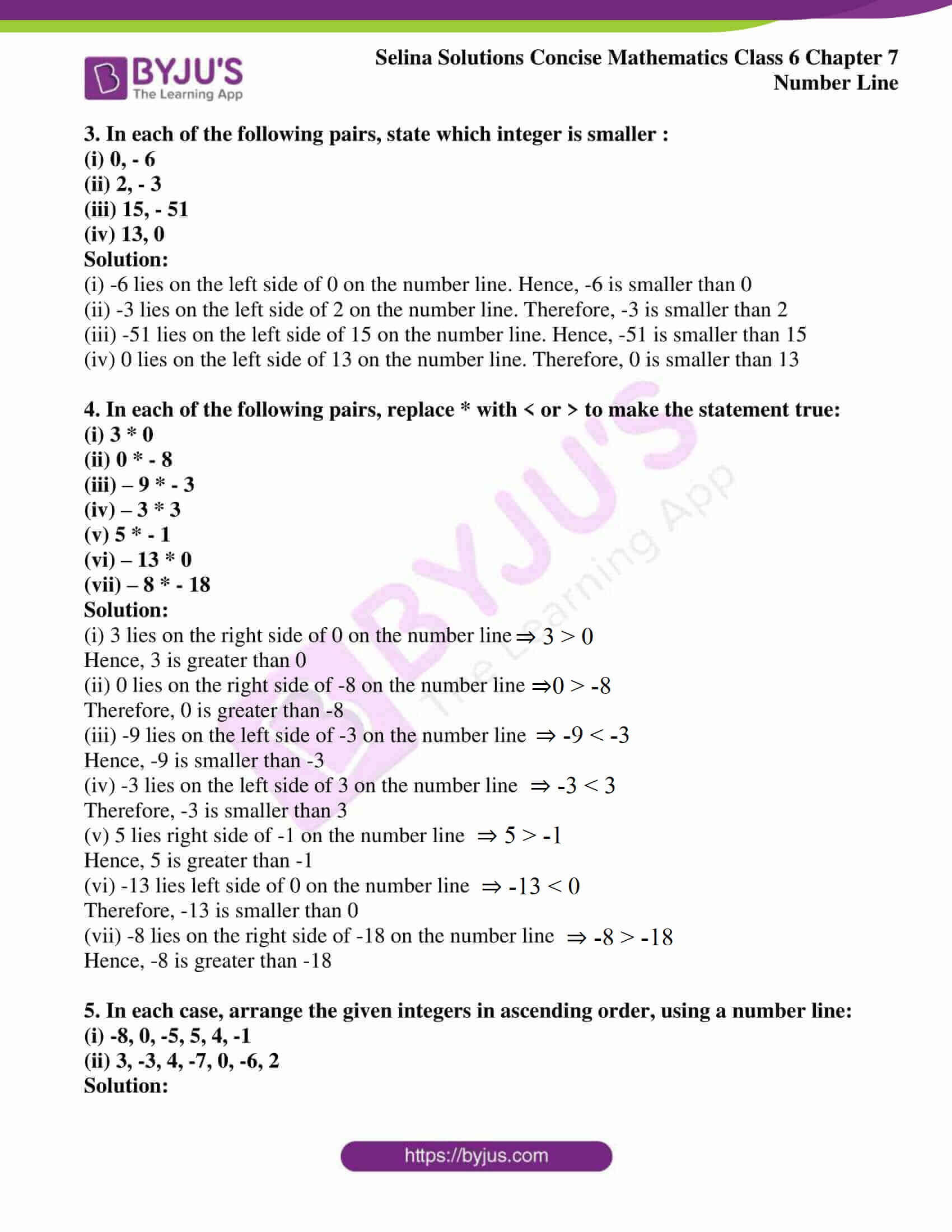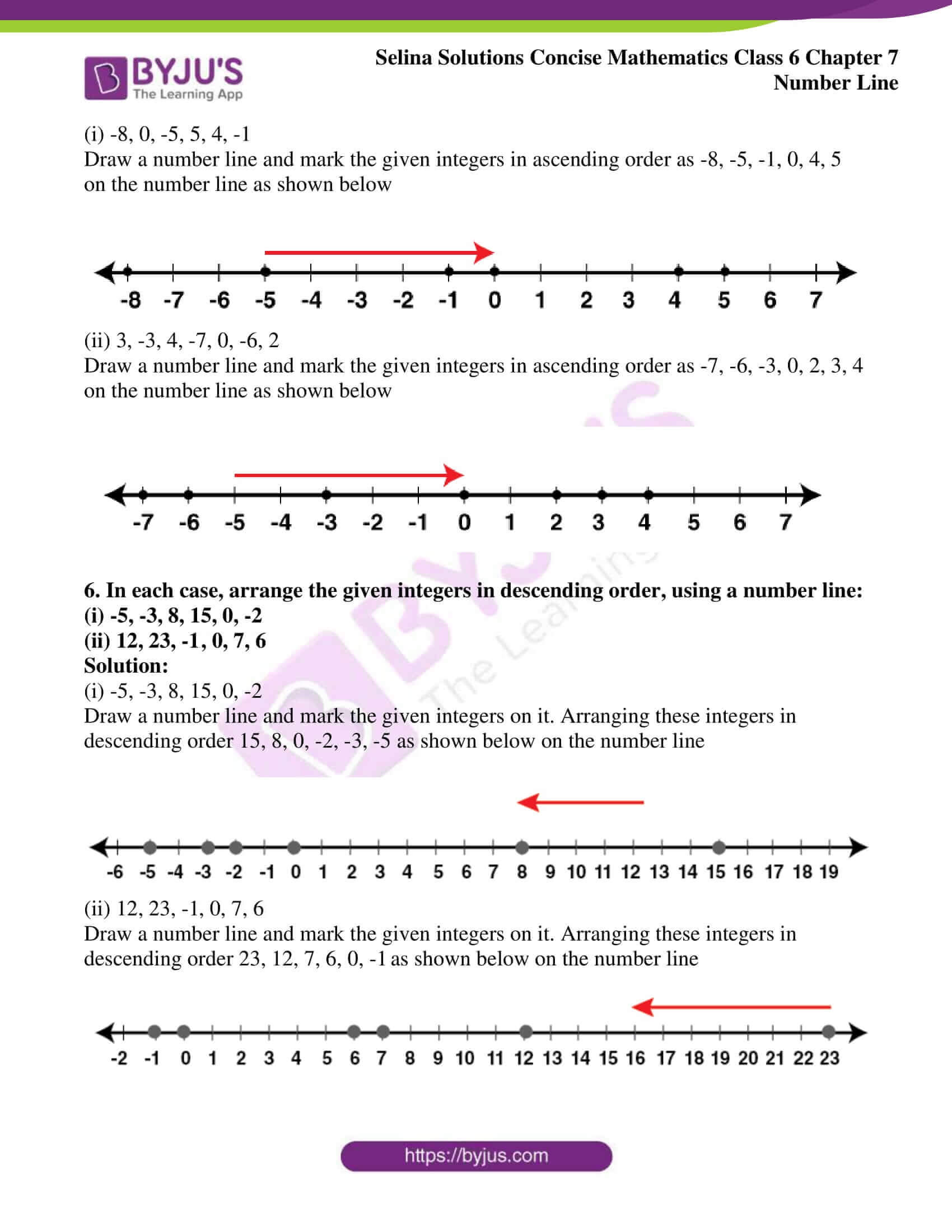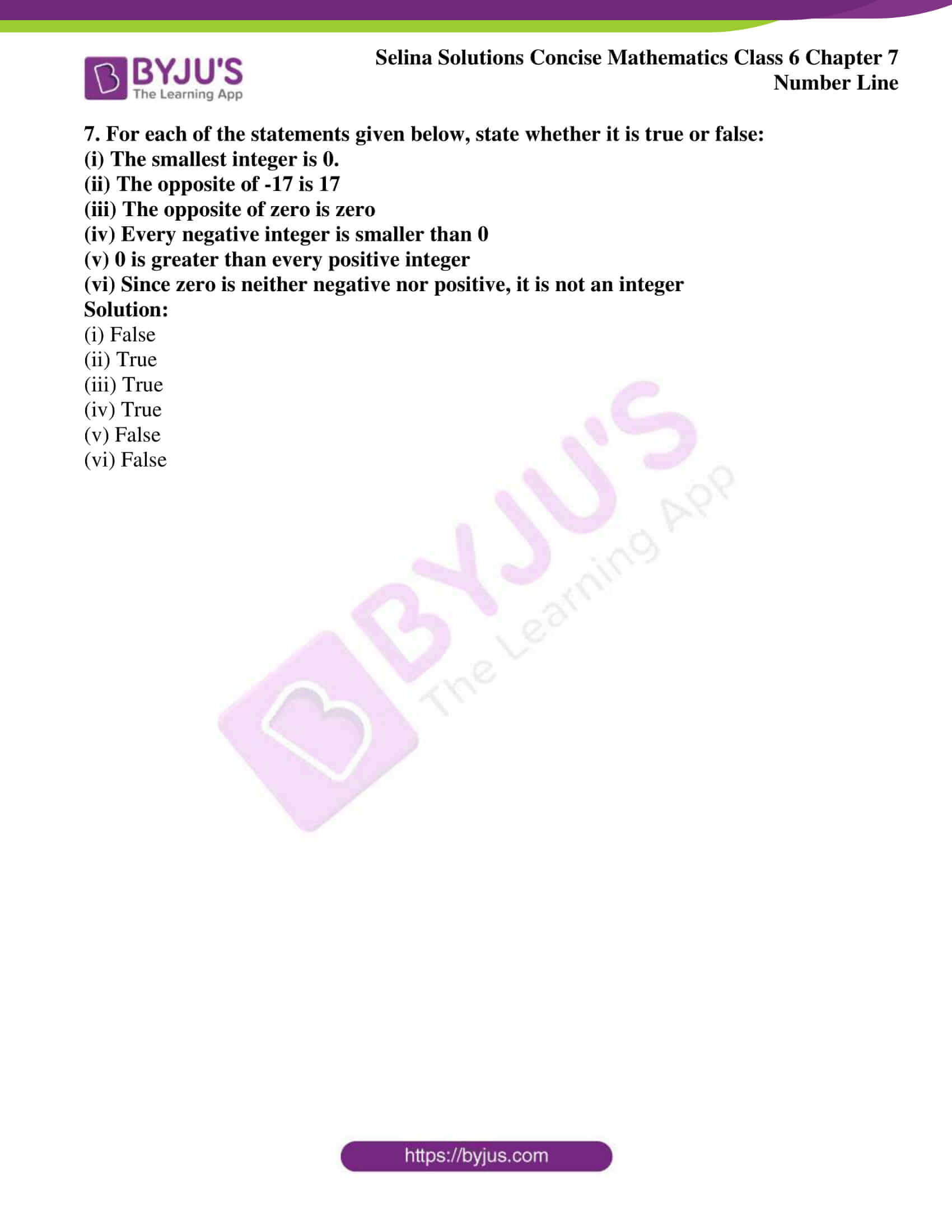### Access another exercise of Selina Solutions Concise Mathematics Class 6 Chapter 7: Number Line

Exercise 7(B) Solutions

### Access Selina Solutions Concise Mathematics Class 6 Chapter 7: Number Line Exercise 7(A)

#### Exercise 7(A) page no: 55

1. Fill in the blanks, using the following number line: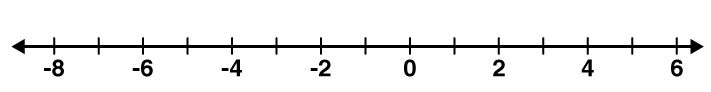(i) An integer, on the given number line, is ………. than every number on its left.

(ii) An integer on the given number line is greater than every number to its ………..

(iii) 2 is greater than -4 implies 2 is to the ……….. of -4.

(iv) -3 is …………. than 2 and 3 is ………… than -2.

(v) -4 is ……….. than -8 and 4 is ……….. than 8.

(vi) 5 is ………….. than 2 and -5 is ………… than -2.

(vii) -6 is …………. than 3 and the opposite of -6 is …………. than opposite of 3.

(viii) 8 is ………….. than -5 and -8 is ……………. than 5.

Solution:

(i) An integer, on the given number line, is greater than every number on its left.

(ii) An integer, on the given number line, is greater than every number to its left.

(iii) 2 is greater than -4 implies 2 is on the right of -4

(iv) -3 is less than 2 and 3 is greater than -2

(v) -4 is greater than -8 and 4 is lessthan 8

(vi) 5 is greater than 2 and -5 is less than -2

(vii) -6 is less than 3 and the opposite of -6 is greater than opposite of 3

(viii) 8 is greater than -5 and -8 is less than 5

2. In each of the following pairs, state which integer is greater :

(i) – 15, – 23

(ii) – 12, 15

(iii) 0, 8

(iv) 0, – 3

Solution:

(i) -15, -23

-15 lies on the right side of -23 on the number line. Therefore, -15 is greater than -23

(ii) -12, 15

15 lies on the right side of -12 on the number line. Hence, 15 is greater than -12

(iii) 0, 8

8 lies on the right side of 0 on the number line. Therefore, 8 is greater than 0

(iv) 0, -3

0 lies on the right side of -3 on the number line. Hence, 0 is greater than -3

3. In each of the following pairs, state which integer is smaller :

(i) 0, – 6

(ii) 2, – 3

(iii) 15, – 51

(iv) 13, 0

Solution:

(i) -6 lies on the left side of 0 on the number line. Hence, -6 is smaller than 0

(ii) -3 lies on the left side of 2 on the number line. Therefore, -3 is smaller than 2

(iii) -51 lies on the left side of 15 on the number line. Hence, -51 is smaller than 15

(iv) 0 lies on the left side of 13 on the number line. Therefore, 0 is smaller than 13

4. In each of the following pairs, replace * with < or > to make the statement true:

(i) 3 * 0

(ii) 0 * – 8

(iii) – 9 * – 3

(iv) – 3 * 3

(v) 5 * – 1

(vi) – 13 * 0

(vii) – 8 * – 18

Solution:

(i) 3 lies on the right side of 0 on the number line ⇒ 3 > 0

Hence, 3 is greater than 0

(ii) 0 lies on the right side of -8 on the number line ⇒ 0 > -8

Therefore, 0 is greater than -8

(iii) -9 lies on the left side of -3 on the number line ⇒ -9 < -3

Hence, -9 is smaller than -3

(iv) -3 lies on the left side of 3 on the number line ⇒ -3 < 3

Therefore, -3 is smaller than 3

(v) 5 lies right side of -1 on the number line ⇒ 5 > -1

Hence, 5 is greater than -1

(vi) -13 lies left side of 0 on the number line ⇒ -13 < 0

Therefore, -13 is smaller than 0

(vii) -8 lies on the right side of -18 on the number line ⇒ -8 > -18

Hence, -8 is greater than -18

5. In each case, arrange the given integers in ascending order, using a number line:

(i) -8, 0, -5, 5, 4, -1

(ii) 3, -3, 4, -7, 0, -6, 2

Solution:

(i) -8, 0, -5, 5, 4, -1

Draw a number line and mark the given integers in ascending order as -8, -5, -1, 0, 4, 5 on the number line as shown below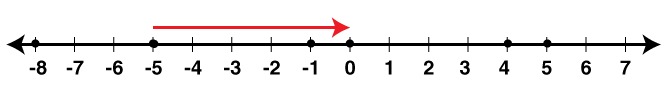(ii) 3, -3, 4, -7, 0, -6, 2

Draw a number line and mark the given integers in ascending order as -7, -6, -3, 0, 2, 3, 4 on the number line as shown below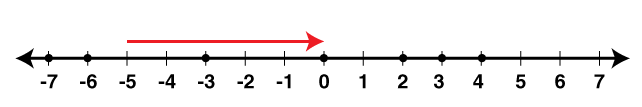6. In each case, arrange the given integers in descending order, using a number line:

(i) -5, -3, 8, 15, 0, -2

(ii) 12, 23, -1, 0, 7, 6

Solution:

(i) -5, -3, 8, 15, 0, -2

Draw a number line and mark the given integers on it. Arranging these integers in descending order 15, 8, 0, -2, -3, -5 as shown below on the number line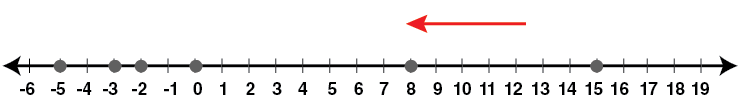(ii) 12, 23, -1, 0, 7, 6

Draw a number line and mark the given integers on it. Arranging these integers in descending order 23, 12, 7, 6, 0, -1 as shown below on the number line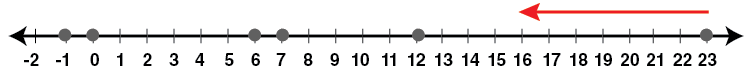7. For each of the statements given below, state whether it is true or false:

(i) The smallest integer is 0.

(ii) The opposite of -17 is 17

(iii) The opposite of zero is zero

(iv) Every negative integer is smaller than 0

(v) 0 is greater than every positive integer

(vi) Since zero is neither negative nor positive, it is not an integer

Solution:

(i) False

(ii) True

(iii) True

(iv) True

(v) False

(vi) False